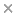BaseSpace
Correlation Engine 2.0Search sequence regions

###### filter terms:
Sizes of these terms reflect their relevance to your search.

When the outcome is binary, psychologists often use nonlinear modeling strategies such as logit or probit. These strategies are often neither optimal nor justified when the objective is to estimate causal effects of experimental treatments. Researchers need to take extra steps to convert logit and probit coefficients into interpretable quantities, and when they do, these quantities often remain difficult to understand. Odds ratios, for instance, are described as obscure in many textbooks (e.g., Gelman & Hill, 2006, p. 83). I draw on econometric theory and established statistical findings to demonstrate that linear regression is generally the best strategy to estimate causal effects of treatments on binary outcomes. Linear regression coefficients are directly interpretable in terms of probabilities and, when interaction terms or fixed effects are included, linear regression is safer. I review the Neyman-Rubin causal model, which I use to prove analytically that linear regression yields unbiased estimates of treatment effects on binary outcomes. Then, I run simulations and analyze existing data on 24,191 students from 56 middle schools (Paluck, Shepherd, & Aronow, 2013) to illustrate the effectiveness of linear regression. Based on these grounds, I recommend that psychologists use linear regression to estimate treatment effects on binary outcomes. (PsycInfo Database Record (c) 2021 APA, all rights reserved).

### Citation

Robin Gomila. Logistic or linear? Estimating causal effects of experimental treatments on binary outcomes using regression analysis. Journal of experimental psychology. General. 2021 Apr;150(4):700-709

PMID: 32969684

View Full Text